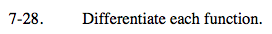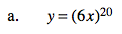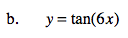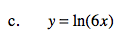### Home > CALC > Chapter 7 > Lesson 7.1.3 > Problem7-28

7-28.
1. Differentiate each function. Homework Help ✎

1. y = (6x)20

2. y = tan(6x)

3. y = ln(6x)Chain rule.
or rewrite as y = 620x20 and use the Power Rule.Recall that the derivative of y = tanx is y' = sec2x..Do you see the pattern?
Notice that all three of these problems ask you to differentiate composite functions with an 'inner' function that has a coefficient of 6.
Compare and contrast their derivatives.